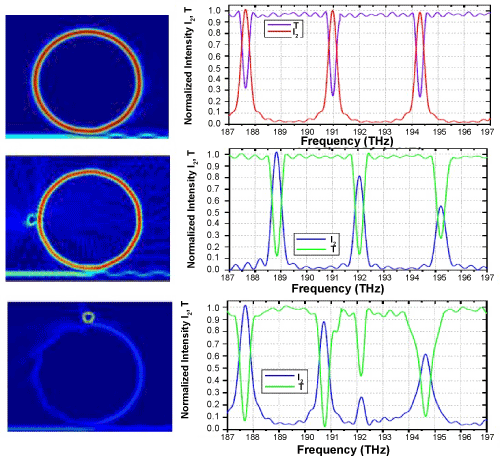Figure 3: Light intensity in frequency-domain in microring sensor (R=10 μm,dR=1μm, n=1.46). First row: (left) intensity of light with frequency f=191 THz, (right) spectrum of nomalized intensity inside cavity I2 (red), and transmission T (purple). Second row: (left) light intensity f=192 THz, for a single SO with r=1 μm, n=2.0, right: spectrum I2 (blue), transmission T (green). Third row:(left) light intensity f=192.2 THz (cavity detuned), for a single SO with r=1 μm,nSO=3.0, I2 (blue) and transmission T (green).
Goto home»